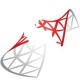# Table Sizes

There’s no simple way in SQL Server to see the sizes of all the tables and their indexes.  Even seeing a single table’s size through SSMS can take a while.  The solution below is grabbed from statistics, so there’s no guarantee you’re getting exact numbers.  However, exact numbers don’t tend to matter, as you care more that the table is about 1 GB, not that it’s exactly 998 MB.

There are variations of this code floating all over the place, and I tweaked the results to be in the easiest to read format possible.

The only issue I have with this script is that you have to scroll to the next-to-the-last line to filter which tables it’s pulling. Yes, I’m picky, and I don’t like to scroll to see things that change.

```SELECT Database_ID = DB_ID()
, Database_Name = DB_NAME()
, Schema_Name = a3.name
, TableName = a2.name
, TableSize_MB = (a1.reserved + ISNULL(a4.reserved,0)) / 128
, RowCounts = a1.rows
, DataSize_MB = a1.data / 128
, IndexSize_MB = (CASE WHEN (a1.used + ISNULL(a4.used,0)) > a1.data
THEN (a1.used + ISNULL(a4.used,0)) - a1.data
ELSE 0
END) /128
, Free_MB = (CASE WHEN (a1.reserved + ISNULL(a4.reserved,0)) > a1.used
THEN (a1.reserved + ISNULL(a4.reserved,0)) - a1.used
ELSE 0
END) / 128
FROM (SELECT ps.object_id
, [rows] = SUM(CASE
WHEN (ps.index_id < 2) THEN row_count
ELSE 0
END)
, reserved = SUM(ps.reserved_page_count)
, data = SUM(CASE
WHEN (ps.index_id < 2)
THEN (ps.in_row_data_page_count + ps.lob_used_page_count + ps.row_overflow_used_page_count)
ELSE (ps.lob_used_page_count + ps.row_overflow_used_page_count)
END)
, used = SUM (ps.used_page_count)
FROM sys.dm_db_partition_stats ps
GROUP BY ps.object_id) AS a1
INNER JOIN sys.all_objects a2  ON a1.object_id = a2.object_id
INNER JOIN sys.schemas a3 ON a2.schema_id = a3.schema_id
LEFT JOIN (SELECT it.parent_id
, reserved = SUM(ps.reserved_page_count)
, used = SUM(ps.used_page_count)
FROM sys.dm_db_partition_stats ps
INNER JOIN sys.internal_tables it ON it.object_id = ps.object_id
WHERE it.internal_type IN (202,204)
GROUP BY it.parent_id) AS a4 ON a4.parent_id = a1.object_id
WHERE a2.type <> 'S' and a2.type <> 'IT'
--AND a2.name IN ('spt_values')
ORDER BY a1.reserved desc```

## 2 thoughts on “Table Sizes”

1.Richard Noel says:

•Steve Hood says: